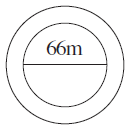# A circular flower bed is surrounded by a path 4 m wide. The diameter of the flower bed is 66 m. What is the area of this path? (π = 3.14)Given

From the given details we get to know that

Diameter of the flower bed = 66 m

Width of path =4m

Find out

We have to determine the area of this path

Solution

We know that

Diameter of the flower bed = 66 m

Radius of the flower bed = d/2

= 66/2

= 33 m

Area of flower bed = πr2

= 3.14 × 332

= 3.14 × 1089

= 3419.46 m

So, radius of flower bed and path together = 33 + 4 = 37 m

Area of the flower bed and path together = πr2

= 3.14 × 372

= 3.14 × 1369

= 4298.66 m

Area of the path = Area of the flower bed and path together – Area of flower bed

= 4298.66 – 3419.46

= 879.20 m2

Area of the path = 879.20 m2(0)(0)